# Self induction

Consider a coil connected to a battery through a key. When the circuit is switched on the current starts growing through the coil and very soon acquires its maximum value. During its growth it produces growing magnetic fields which is also linked with the coil. Due to such a field EMF in induced in the coil which according to lenz’s law oppose the growth of current. This EMF is known as back EMF.

The phenomenon due to which back EMF is induced is a coil due to the varying currents flowing through the coil itself is known as self induction.

ɸ  x  I

ɸ  x  L I

is a constant known as “ coefficient of self Induction.” It is also known as self inductance as simply the “ inductance”

The coil itself may be called as inductance .

Differentiating,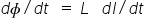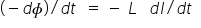-ve sign shows that

e opposes growing current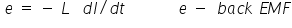Unit of induction Henry (H)

Self Induction of a coil is said to be 1 henry  if  1 volt of EMF is induced in it when current through it changes at a rate of 1 ampere/sec.

Expression for self inductances

ɸ = N.BA= nl μon I A

ɸ = μon2 l A I

but   ɸ = L I

so L =μn2 lA

Self inductance of coil depends upon physical parameter and is independent of the current passing there it as well as flue linked with it.

Combination of Inductances.

1.Parallel combination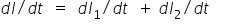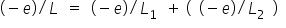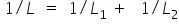2. Series Combination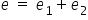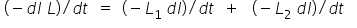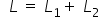Related Keywords